## Modular Equation

The modular equation of degreegives an algebraic connection of the form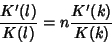(1)

between the Transcendental Complete Elliptic Integrals of the First Kind with moduli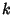and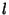. Whenandsatisfy a modular equation, a relationship of the form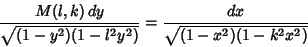(2)

exists, and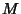is called the Modular Function Multiplier. In general, if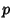is an Odd Prime, then the modular equation is given by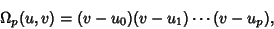(3)

where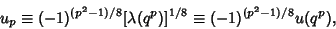(4)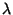is a Elliptic Lambda Function, and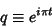(5)

(Borwein and Borwein 1987, p. 126). An Elliptic Integral identity gives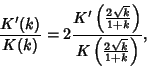(6)

so the modular equation of degree 2 is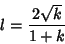(7)

which can be written as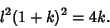(8)

A few low order modular equations written in terms ofandare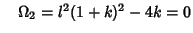(9)(10)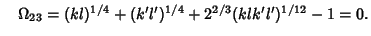(11)
In terms of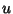and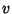,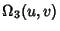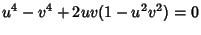(12)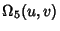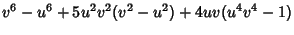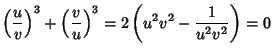(13)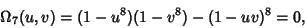(14)

where(15)

and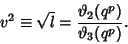(16)

Here,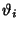are Theta Functions.

A modular equation of degree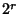for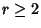can be obtained by iterating the equation for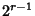. Modular equations for Primefrom 3 to 23 are given in Borwein and Borwein (1987).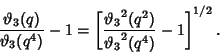(17)

Cubic identities include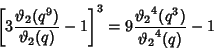(18)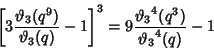(19)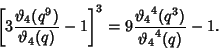(20)

A seventh-order identity is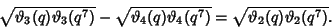(21)

From Ramanujan (1913-1914),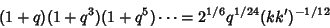(22)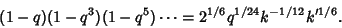(23)

References

Borwein, J. M. and Borwein, P. B. Pi & the AGM: A Study in Analytic Number Theory and Computational Complexity. New York: Wiley, pp. 127-132, 1987.

Hanna, M. The Modular Equations.'' Proc. London Math. Soc. 28, 46-52, 1928.

Ramanujan, S. Modular Equations and Approximations to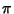.'' Quart. J. Pure. Appl. Math. 45, 350-372, 1913-1914.